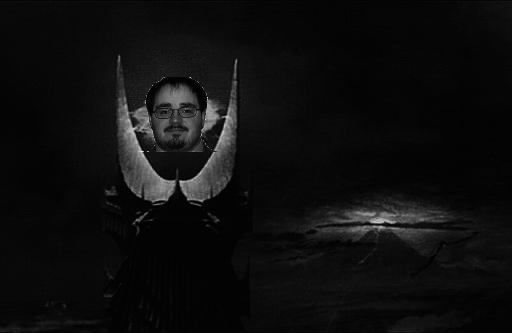# CPSC120AFundamentals of Computer Science I

## Scientific Assignment 8

Use Python to Create Facial Recognition Software.

There are many uses for an algorithm that can detect faces. For example, some cameras use facial detection to determine where to focus the camera. Facebook uses facial detection to determine whether there is someone to tag in the picture. While fully functioning face detection algorithms can be very complicated, we can perform a simple version of face detection using our PPM images.

### Details

Write a function called find_face(template_image_name, image_name), which takes two strings as parameters. The first string is the name of a template image, while the second string is the name of a normal image. Both of these images should be in PPM format, and should be greyscale images. This function should return a tuple, the location the upper-left corner where the face was found in image_name.

The template image will look like follows:

it is an image that describes what a face looks like.

An example image looks like this:Which is just a "normal" image.

Your job is to find the location that best matches the template image within the normal image.

You are going to determine this by computing the error between the specified template image and the appropriate pixels in the normal image. You can compute error by computing the mean squared error between the two images. Mean squared error is simply the average of the squared differences between the pixel values. Consider the following example:

Where image 1 is

 (0, 1, 2) (255, 255, 255) (255, 255, 255) (3, 4, 5)
and Image 2 is
 (6, 7, 8) (255, 255, 255) (0, 0, 0) (255, 255, 255) (255, 255, 255) (9, 10, 11) (255, 255, 255) (0, 0, 0) (0, 0, 0) 255, 255, 255) (0, 0, 0) (255, 255, 255) (255, 255, 255) (0, 0, 0) (255, 255, 255) (0, 0, 0)

If we were comparing starting at row = 0, col = 0 we are comparing the pixels $$(0, 1, 2)$$ and $$(6, 7, 8)$$ the error of the first pixel is: $p00\_error = \frac{(0 - 6) ^ 2 + (1 - 7) ^ 2 + (2 - 8) ^ 2}{3} = 36$ However comparing the next pixels ($$(255, 255, 255)$$ and $$(255, 255, 255)$$) is: $p01\_error = \frac{(255 - 255) ^ 2 + (255 - 255) ^ 2 + (255 - 255) ^ 2}{3} = 0$ Which when extended across the total overlap between the images, the mean squared error gives: $\frac{p00\_error + p01\_error + p10\_error + p11\_error}{4} = 18$

However, if we were comparing starting at row = 0, col = 1 ($$(0, 1, 2)$$ and $$(255, 255, 255)$$): $p00\_error = \frac{(0 - 255) ^ 2 + (1 - 255) ^ 2 + (2 - 255) ^ 2}{3} = 64516.666666666664$ and the next comparison ($$(255, 255, 255)$$ and $$(0, 0, 0)$$): $p01\_error = \frac{(255 - 0) ^ 2 + (255 - 0) ^ 2 + (255 - 0) ^ 2}{3} = 195075$ and the mean squared error between the two: $\frac{p00\_error + p01\_error + p10\_error + p11\_error}{4} = 95654.75$

You should assume that there is a face in the image. You will check all possible locations the face can be in. The location that has the face will be the one with the lowest mean squared error.

You may use the PPM module provided in lecture 27 to read in your PPM images. Once you successfully find the face in the first image, you can try your luck with this image:### Hacker Prompt

1. Outline the Face: Once you have found the face, you'll want some mechanism to see where that face actually is. Modify your code so that once it finds the face, it outlines the face with a red box.
2. Where's Waldo: We have been working assuming that the images were in gray scale. Alter your code so that it also works for colored template images.
3. Scaled template: You are assuming that the template exists at full size in the normal image. This won't always be the case. Add scaling of the template image to the code. You should scale the template by a factor of 2 until you have a 2 x 2 template image.

## Submission

Your code should conform to the course’s code conventions and will be graded according the the course’s programming assignment rubric. Submit your program as a .py file on the course Inquire page before class on Friday November 21th.